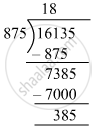# Divide, and find out the quotient and remainder. Check your answer. 16135 ​÷ 875 - Mathematics

Fill in the Blanks

16135 ​÷ 875

#### SolutionQuotient = 18 and Remainder = 385
Check: Divisor × Quotient + Remainder =875 × 18 + 385
= 16135
= Dividend
∴ Dividend= Divisor × Quotient +Remainder

Is there an error in this question or solution?

#### APPEARS IN

RS Aggarwal Class 6 Mathematics
Chapter 3 Whole Numbers
Exercise 3E | Q 2.6 | Page 56